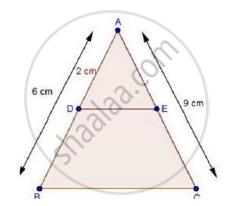Share

# In δAbc, D and E Are Points on the Sides Ab and Ac Respectively Such that De || Bc If Ad = 2 Cm, Ab = 6 Cm and Ac = 9 Cm, Find Ae. - CBSE Class 10 - Mathematics

ConceptBasic Proportionality Theorem Or Thales Theorem

#### Question

In ΔABC, D and E are points on the sides AB and AC respectively such that DE || BC

If AD = 2 cm, AB = 6 cm and AC = 9 cm, find AE.

#### SolutionWe have,

AD = 2 cm, AB = 6 cm

∴ DB = AB – AD

= 6 – 2

⇒ DB = 4 cm

And, DE || BC

Therefore, by basic proportionality theorem, we have,

"AD"/"DB"="AE"/"EC"

Taking reciprocal on both sides, we get,

"DB"/"AD"="EC"/"AE"

4/2="EC"/"AE"

Adding 1 on both sides, we get

4/2+1="EC"/"AE"+1

rArr(4+2)/2=("EC"+"AE")/"AE"

rArr6/2="AC"/"AE"         [∵ EC + AE = AC]

rArr6/2=9/"AE"              [∵ AC = 9cm]

"AE"=(9xx2)/6

⇒ AE = 3 cm

Is there an error in this question or solution?

#### Video TutorialsVIEW ALL 

Solution In δAbc, D and E Are Points on the Sides Ab and Ac Respectively Such that De || Bc If Ad = 2 Cm, Ab = 6 Cm and Ac = 9 Cm, Find Ae. Concept: Basic Proportionality Theorem Or Thales Theorem.
S